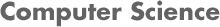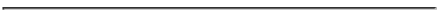### CS 548 KNOWLEDGE DISCOVERY AND DATA MINING - Fall 2018 Homework Assignments

#### PROF. CAROLINA RUIZ### General advice to do well in this course:

1. Read the textbook. This is really important. The textbook is available on Reserved in the WPI Gordon Library under "CS548". This means you can go to the library and ask at the library front desk to borrow the book for a period of 2 hours.

2. Attend the lectures and participate in class.

3. Class participation (including participating during the lectures, volunteering to solve problems on the whiteboard, posting good questions and good answers on the Cavas Discussion Board) count towards your course grade.

4. Read the textbook and the materials assigned for each class (see links on the course schedule webpage) BEFORE each lecture. This is really important.

5. Work on every part of the project yourself so that you know the content first hand and can answer the questions on the test.

6. Start working on the projects as soon as they are posted - they take longer than what you think at first.

7. Use the guidelines on https://web.cs.wpi.edu/~ruiz/KDDRG/Resources/QuizzesExams/cs548_topics_quizzes.html (which are linked in several places on the course schedule) to prepare for the tests.

8. Attend both the Professor's and the TA's office hours, ask in class and/or post questions on the course discussion board if you have any questions about the material.

9. Pay attention to the Showcase presentations and ask questions. The tests will include questions about the showcases.

### Homework Assignments:

Be prepared for possible pop-quizzes during class based on the homework assignments.

• BEFORE next classes on Clustering (Thu Nov. 8th and Tu Nov 13th):
Note: No need to submit solutions to this homework but be prepared to discuss your work during class.

1. Solve the problems on Prof. Ruiz's Hierarchical Clustering Handout BEFORE class on Thursday, Nov. 8th.

2. Study again Section 2.4 of the textbook on "Measures of Similarity and Dissimilarity" (except for Section 2.4.7 and 2.4.8). As a result, you should be familiar with the following concepts:
• Properties of distance metrics (positivity, symmetry and triangle inequality).
• Specific formulas and intuition behind the following distance metrics, and their pros and cons in practice:
• Euclidean, Manhattan, Hamming, Minkowski (with p ≥ 1), Mahalanobis.
• Specific formulas and intuition behind the following similarity coeffients, and their pros and cons in practice:
• Simple Matching Coefficient (SMC), Jaccard Coefficient, Cosine Similarity, Correlation, Mutual Information.
• Issues in proximity calculations; using weights; and selecting the right proximity measure.

3. Study Chapter 7 of the textbook on "Cluster Analysis: Basic Concepts and Algorithms".

4. Study all the materials posted on the course Lecture Notes, especially those marked with "**":

5. Answer the questions on clustering and solve exercises posted on the Quiz/Exam Topics and Sample Questions.

• BEFORE classes on Artificial Neural Networks and Deep Learning:
Note: No need to submit solutions to this homework but be prepared to discuss your work during class.

1. Study Section 4.7 Artificial Neural Networks and Sectio 4.8 Deep Learning of the textbook in great detail.

2. Study all the materials posted on the course Lecture Notes, especially those marked with "**":

• BEFORE class on Tuesday, Oct. 9, 2018:
Note: No need to submit solutions to this homework but be prepared to discuss your work during class.

1. Let M be a model and S be a test set of size n=100, where each data instance in S was drawn independently of M and independently of each other. Assume that the classification error of M over S is 0.28 (i.e., M's classification accuracy over S is 72%). Calculate the 95% confidence interval for this error. What can you say about the error of M over the entire set of data instances in the domain based on this confidence interval? Show your work.

2. Same question 1 above but now for the 80% confidence interval.

3. Let M1 and M2 be models and S1 and S2 be test sets of size n1=100 and n2=60 respectively, where each data instance in Si (for i = 1, 2) was drawn independently of Mi and independently of each other. Assume that the classification error of M1 over S1 is 0.28 (i.e., M1's classification accuracy over S1 is 72%) and the classification error of M2 over S2 is 0.19 (i.e., M2's classification accuracy over S2 is 81%). Determine whether or not M2 is statistically significantly better than M1 for p < 0.05. Show your work.

4. Same question 3 above but now for p < 0.2.

5. Same question 3 above but now assuming that n1 = 1000 and n2 = 600.

• BEFORE class on Tuesday, Oct. 2, 2018:
Note: No need to submit solutions to this homework but be prepared to discuss your work during class and ask questions you have about the material.

Model Evaluation and Model Comparison:

1. Prof. Ruiz's Lecture Notes: Model Evaluation.
2. Tan, Steinbach, Karpatne, Kumar's Textbook: Sect. 3.4-3.9.
3. Weka's Textbook: Chapter 5 and Chapter 5 Slides.
Very important section: Evaluating Numeric Predictions.
4. Prof. Ruiz's Lecture Notes: Confidence Intervals.

• BEFORE class on Thursday, Sept. 27, 2018:
Note: No need to submit solutions to this homework but be prepared to discuss your work during class.

1. Study linear regression, model trees and regression trees using the Weka book: Sections 3.2, 3.3, 4.6 and
• If you have Witten's, Frank's, and Hall's 3rd edition of the book (available on reserve in the WPI Library under "CS548"): Section 6.6 (Numeric Predictions with Local Linear Models).
• If you have Witten's, Frank's, Hall's and Pal's 4th edition of the book: Section 7.3 (Numeric Predictions with Local Linear Models).

2. Study all the materials posted on the course Lecture Notes under: especially those marked with "**". You should know the algorithms to construct regression trees and model trees very well, and be able to use these algorithms to construct trees from data by hand See examples provided in the Lecture Notes linked above.

3. IMPORTANT: Answer the questions on the Handout: Model and Regression Trees. Be prepared to present and discuss your answers in class on Thursday.

4. Experiment with Regression Trees and Model Trees in Weka:
1. Use a dataset of your choice, for example diabetes.arff.
2. Go to the "Classify" tab. Choose a continuous attribute as the target, for example "preg".
3. Click on "Choose" and pick Trees and then M5P.
4. Click on "Start" and look at the results.
5. Right-click on the Results list (on the left panel) and select "Visualize tree".
6. Right-click on the top "M5P -M 4 0" bar and learn about the M5P parameters. Experiment with changing parameter values and looking at the resulting trees. Record any observations you make.

5. Study linear regression on Appendix D (online) of the textbook.